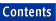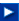Apache C++ Standard Library User's Guide

## 28.3 Format Control Using the Stream's Format State

### 28.3.1 Format Parameters

Associated with each stream are a number of format state variables that control the details of formatting and parsing. Format state variables are classes inherited from a stream's base class, either ios_base or the class template basic_ios. There are two kinds of format parameters: those that can have an arbitrary value, and those that can have only a few different values.

#### 28.3.1.1 Parameters That Can Have an Arbitrary Value

The value is stored as a private data member in one of the base classes, and set and retrieved through public member functions inherited from that base class. There are three such parameters, described in Table 27:

#### Table 27: Format parameters with arbitrary values

 Access function Defined in base class Effect Default width() ios_base Minimal field width 0 precision() ios_base Precision of floating point values 6 fill() basic_ios Fill character for padding The space character

#### 28.3.1.2 Parameters That Can Have Only a Few Different Values

Typically, these would have just two or three different values. These parameters are represented by one or more bits in a data member of type std::iosbase::fmtflags in class ios_base. These are usually called format flags. You can set format flags using the setf() function in class ios_base, clear them using unsetf(), and retrieve them through the flags() function.

Some format flags are grouped because they are mutually exclusive; for example, output within an output field can be adjusted to the left or to the right, or to an internally specified adjustment. One and only one of the corresponding three format flags, left, right, or internal, can be set. (Iostreams does not prevent you from setting other invalid combinations of these flags, however.) If you want to set one of these bits to 1, you need to set the other two to 0. To make this easier, there are bit groups whose main function is to reset all bits in one group. The bit group for adjustment is adjustfield, defined as left | right | internal.

Table 28 gives an overview of all format flags and their effects on input and output operators. (For details on how the format flags affect input and output operations, see the Apache C++ Standard Library Reference Guide entry for ios_base.) The first column below, format flag, lists the flag names; for example, showpos stands for std::ios_base::showpos. The group column lists the name of the group for flags that are mutually exclusive. The third column gives a brief description of the effect of setting the flag. The stdio column refers to format characters used by the C functions scanf() or printf() that have the same or similar effect. The last column, default, lists the setting that is used if you do not explicitly set the flag.

#### Table 28: Flags and their effects on operators

 Format flag Group Effect stdio Default adjustfield Adds fill characters to certain generated output for adjustment: left (no bits set) left left - right right 0 internal Adds fill characters at designated internal point none basefield Converts integer input or generates integer output in: %i dec dec decimal base %d,%u oct octal base %o,%O hex hexadecimal base %x,%X floatfield Generates floating point output: %g,%G fixed fixed in fixed-point notation %f scientific in scientific notation %e,%E boolalpha Inserts and extracts bool values in alphabetic format 0 showpos Generates a + sign in non-negative generated numeric output + 0 showpoint Always generates a decimal-point in generated floating-point output .n (n indicates number of decimals) 0 showbase Generates a prefix indicating the numeric base of a generated integer output # 0 skipws Skips leading white space before certain input operations none 1 unitbuf Flushes output after each formatting operation none 0 uppercase Replaces certain lowercase letters with their uppercase equivalents in generated output %X %E %G 0

The effect of setting a format parameter is usually permanent; that is, the parameter setting is in effect until the setting is explicitly changed. The only exception to this rule is the field width. The width is automatically reset to its default value, 0, after each input or output operation that uses the field width. Here is an example:

```int i; char* s;
std::cin  >> setw(10) >> i >> s;                              //1
std::cout << setw(10) << i << s;                              //2
```
 //1 Extracting an integer is independent of the specified field width. The extractor for integers always reads as many digits as belong to the integer. As extraction of integers does not use the field width setting, the field width of 10 is still in effect when a character sequence is subsequently extracted. At most 10 characters are extracted in this case. After the extraction, the field width is reset to 0. //2 The inserter for integers uses the specified field width and fills the field with padding characters if necessary. After the insertion, it resets the field width to 0. Hence, the subsequent insertion of the string does not fill the field with padding characters for a string with less than 10 characters.

NOTE -- With the exception of the field width, all format parameter settings are permanent. The field width parameter is reset after each use.

The following code sample shows how you can control formatting by using some of the parameters:

```#include <iostream>

// ...
std::ios_base::fmtflags original_flags = std::cout.flags();   //1
std::cout<< 812<<'|';
std::cout.setf(std::ios_base::left,
std::ios_base::adjustfield);                   //2
std::cout.width(10);                                          //3
std::cout << 813 << 815 << '\n';
std::cout.unsetf(ios_base::adjustfield);                      //4
std::cout.precision(2);
std::cout.setf(std::ios_base::uppercase |
std::ios_base::scientific);                             //5
std::cout << 831.0 << ` ` << 8e2;
std::cout.flags(original_flags);                              //6
```
 //1 Store the current format flag setting, in order to restore it later on. //2 Change the adjustment from the default setting right to left. //3 Set the field width from its default 0 to 10. A field width of 0 means that no padding characters are inserted, and this is the default behavior of all insertions. //4 Clear the adjustment flags. //5 Change the precision for floating-point values from its default 6 to 2, and set yet another couple of format flags that affect floating-point values. //6 Restore the original flags.

The output is:

```812|813       815
8.31E+02 8.00E+02
```

### 28.3.2 Manipulators

Format control requires calling a stream's member functions. Each such call interrupts the respective shift expression. But what if you need to change formats within a shift expression? This is possible in iostreams. In the preceding example, instead of writing:

```std::cout << 812 << '|';
std::cout.setf(std::ios_base::left, std::ios_base::adjustfield);
std::cout.width(10);
std::cout << 813 << 815 << '\n';
```

you can write:

```std::cout << 812 << '|' << std::left << std::setw(10) << 813 << 815
<< std::endl;
```

In this example, objects like left, setw, and endl are called manipulators. There are overloaded versions of the insertion and extraction operators for manipulators, so that manipulators can be extracted from or inserted into a stream together with other objects that have the shift operators defined. (Section 28.3.2 explains in greater detail how manipulators work and how you can implement your own manipulators.)

The effect of a manipulator need not be an actual input to or output from the stream. Most manipulators set just one of the above described format flags, or do some other kind of stream manipulation. For example, an expression like:

```std::cout << std::left;
```

is equivalent to:

```std::cout.setf (std::ios_base::left, std::ios_base::adjustfield);
```

Nothing is inserted into the stream. The only effect is that the format flag for adjusting the output to the left is set.

On the other hand, the manipulator std::endl inserts the newline character to the stream, and flushes to the underlying stream buffer. The expression:

```std::cout << std::endl;
```

is equivalent to:

```(std::cout << '\n').flush();
```

Some manipulators take arguments, like std::setw(int). The setw manipulator sets the field width. The expression:

```std::cout << std::setw(10);
```

is equivalent to:

```std::cout.width(10);
```

In general, you can think of a manipulator as an object you can insert into or extract from a stream, in order to manipulate that stream. Some manipulators can be applied only to output streams, others only to input streams. Most manipulators change format bits only in one of the stream base classes, ios_base or basic_ios. These can be applied to input and output streams.

Table 29 gives an overview of all manipulators defined by iostreams. The first column, Manipulator, lists its name. All manipulators are defined in the namespace ::std. The second column, Use, indicates whether the manipulator is intended to be used with istreams (i), ostreams (o), or both (io). The third column, Effect, summarizes the effect of the manipulator. The last column, Equivalent, lists the corresponding call to the stream's member function.

Note that the second column indicates only the intended use of a manipulator. In many cases, it is possible to apply an output manipulator to an input stream, and vice versa. Generally, this kind of non-intended manipulation is harmless in that it has no effect. For instance, if you apply the output manipulator showpoint to an input stream, the manipulation is simply ignored. However, if you use an output manipulator on a bidirectional stream during input, the manipulation does not affect current input operations, but subsequent output operations.

#### Table 29: Manipulators

 Manipulator Use Effect Equivalent boolalpha io Puts bool values in alphabetic format io.setf(ios_base::boolalpha) dec io Converts integers to/from decimal notation io.setf(ios_base::dec, ios_base::basefield) endl o Inserts newline and flushes buffer o.put(o.widen('\n')); o.flush() ends o Inserts end of string character o.put(o.widen('\0')) fixed o Puts floating point values in fixed-point notation o.setf(ios_base::fixed, ios_base::floatfield) flush o Flushes stream buffer o.flush() hex io Converts integers to/from hexadecimal notation io.setf(ios_base::hex, ios_base::basefield) internal o Adds fill characters at a designated internal point o.setf(ios_base::internal, ios_base::adjustfield) left o Adds fill characters for adjustment to the left o.setf(ios_base::left, ios_base::adjustfield) noboolalpha io Resets the above io.unsetf(ios_base::boolalpha) noshowbase o Resets the above o.unsetf (ios_base::showbase) noshowpoint o Resets the above o.unsetf (ios_base::showpoint) noshowpos o Resets the above o.unsetf (ios_base::showpos) noskipws i Resets the above i.unsetf(ios_base::skipws) nounitbuf o Resets the above o.unsetf(ios_base::unitbuf) nouppercase o Resets the above o.unsetf (ios_base::uppercase) oct io Converts to/from octal notation io.setf(ios_base::oct, ios_base::basefield) resetiosflags (ios_base::fmt flags mask) io Clears ios flags io.setf((ios_base::fmtflags) 0, mask) right o Adds fill characters for adjustment to the right o.setf(ios_base::right, ios_base::adjustfield) scientific o Puts floating point values in scientific notation o.setf(ios_base::scientific, ios_base::floatfield) setbase (int base) io Sets base for integer notation (base = 8, 10, 16) io.setf (base == 8?ios_base::oct: base == 10 ? ios_base::dec : base == 16 ? ios_base::hex : ios_base::fmtflags(0), ios_base::basefield) setfill (char_type c) io Sets fill character for padding io.fill(c) setiosflags (ios_base::fmt flags mask) io Sets ios flags io.setf(mask) setprecision (int n) io Sets precision of floating point values io.precision(n) setw(int n) io Sets minimal field width io.width(n) showbase o Generates a prefix indicating the numeric base of an integer o.setf(ios_base::showbase) showpoint o Always generates a decimal-point for floating-point values o.setf(ios_base::showpoint) showpos o Generates a + sign for non-negative numeric values o.setf(ios_base::showpos) skipws i Skips leading white space i.setf(ios_base::skipws) unitbuf o Flushes output after each formatting operation o.setf(ios_base::unitbuf) uppercase o Replaces certain lowercase letters with their uppercase equivalents o.setf(ios_base::uppercase) ws i Skips white spaces i.ws()